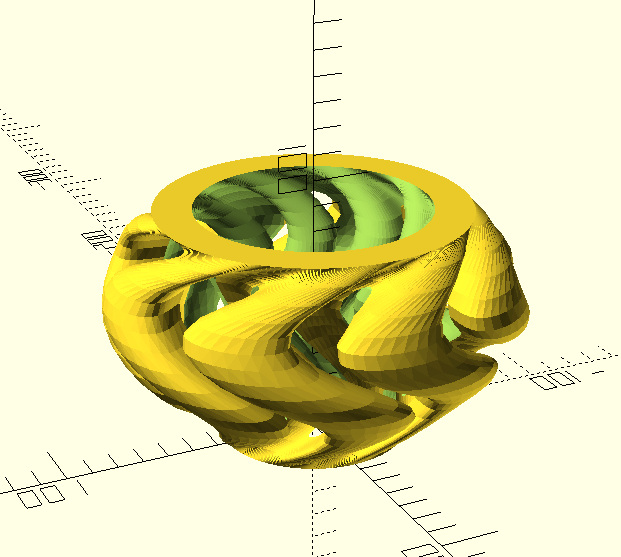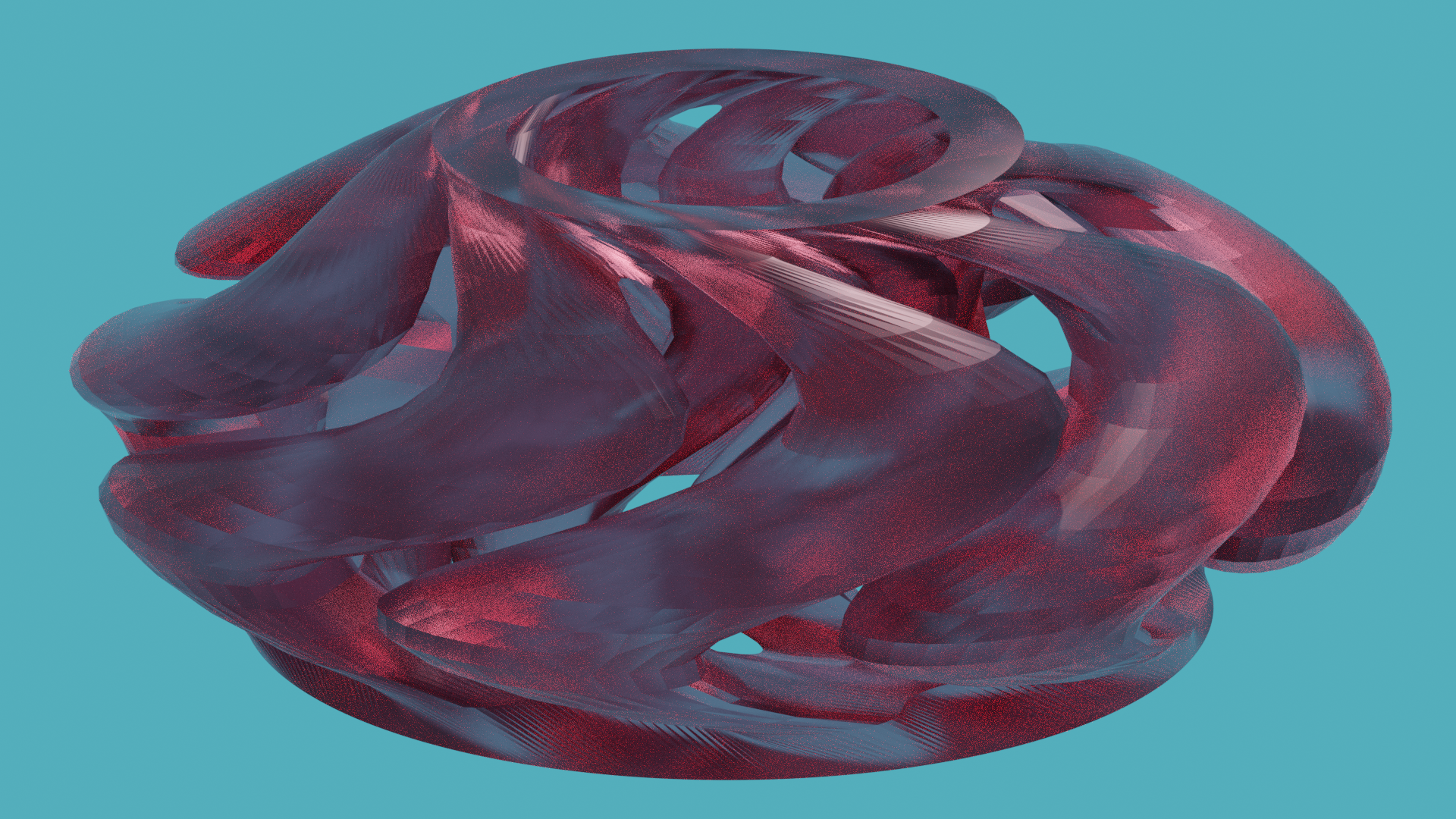For my first project in OpenSCAD I kept it pretty simple and made a simple heart shaped vase utilizing some code from this URL.

While the finished project isn’t spectacular or complex, I did learn a lot about how useful modules are while creating it. I am a computer science major, but OpenSCAD’s syntax was kind of hard to get used to coming from Java. After I figured out where what felt like an infinite number of brackets were supposed to go, I concentrated on learning about modules. Modules are similar to methods in Java, so that part wasn’t too hard. I realize that my code does not adhere to best practice, or even good practice with regard to method re-usability, but the purpose was exploration, not versatility so I think that’s okay.

For future projects I am excited to try using recursion and loops to iterate through and manipulate points more easily to create more complicated or mathematically interesting designs.

Here is my code:

```// The first two heart modules are not my code, they are from:
// The heart_shell module and all other modules are my own.

// Create the 2D heart shape.

rotated_angle = 45;
\$fn = 48;

rotate(-rotated_angle) union() {
square(diameter);
}
}

translate([0, center_offset_y, 0]) union() {
}
}

// Make the first heart shape created hollow.

module heart_shell() {
difference() {
}
};

// Extrude the heart hollow heart shape.
module heart_extrude() {
linear_extrude(height = 15, twist = 75, slices = 20,\$fn = 16) {
heart_shell();
}
}

heart_extrude();

// Creates a base for the heart vase.

```

Disclaimer: I just finished moving for the second time in 2 months after our landlord was unwilling to properly mitigate exposed asbestos…! So I apologize for spending slightly less time on this assignment than I would have otherwise. Anyway…This time around I made a bracelet/bangle object using this Rippled Vase Generator by Fernando Jerez. The shapes generated by this generator are not hollow. To make a bracelet shape, I created another shape with the exact same parameters, shrank the width and height, and took the difference of this and the original shape. Below is a more detailed render of the .STL in Blender.My laptop (desktop isn’t set up yet) did NOT like doing this, but it looks pretty cool.

The result is really interesting to look at! Though I have no idea how one would make it viable for 3D printing. If I were to iterate this I would make sure it was printable and fine-tune the thickness of all of the walls and the size (so that it would fit on a wrist). Adding some kind of closure so that it could open and close would also be required.

The code I used is below. I changed out most of the parameters for the generator and added the additional shape and difference function near the bottom.

```/*

Vase-wave generator/customizer

Fernando Jerez 2017

*/
// Use 'preview' for fast render
part = "preview"; // [preview:Low poly (fast render), print: High detail (slow render, for print)]

// Style / Shape
shape = "bowl"; // [barrel: Penholder/Barrel, vase1:Vase, vase2:Vase 2, glass: Glass, bowl:Bowl, cup: Cup, cone: Cone ]

// Number of ripples
ripples = 8; // [1:15]

// Ripple size
ripple_size = 30; // [10:30]

// Twisting factor
twist = 0.2; // [-1:0.05:1]

// Waving
wave = 30; // [-30:0:30]

/* [Hidden] */
ripple_width = 100/ripples;

altura = 180; // z-steps
step = (part=="preview")?10:1; // resolution in Z (1 is the best and slower)

sides = 360; // Don't touch!

// Calculate....
//points...
puntos = [
for(z = [0:step:altura])
for(i = [0:sides-1])
let(
rot2 = twist*z + wave*sin(z*2),

rgi = ripple_width * sin(z),
giro = rgi * sin(i*ripples*2),

// shape
zrad = (shape=="barrel") ? 1 :
(shape=="vase1") ? 0.7+ 0.4*sin(z*2) :
(shape=="vase2") ? 0.6+ 0.4*pow(sin(z+60),2) :
(shape=="glass") ? 0.6+ 0.4*pow(sin(z*0.7-30),2) :
(shape=="cup") ? 0.65+ 0.4 * cos(z*2) :
(shape=="bowl") ? 0.6+ 0.4 * sin(z*0.5) :
cos(z*0.5),

px = r*cos(i+giro+rot2),
py = r*sin(i+giro+rot2)

)

[px,py,z]
];

// faces...
caras = concat(
[
// Triangle #1
for(z = [0:(altura-1) / step])
for(s = [0:sides-1])
let(
// clockwise from left-down corner
f1 = s + sides*z,
f2 = s + sides*(z+1),
f3 = ((s+1) % sides) + sides*(z+1),
f4 = ((s+1) % sides) + sides*z
)

[f1,f2,f3]

],
[
// Triangle #2
for(z = [0:(altura-1) / step])
for(s = [0:sides-1])
let(
// clockwise from left-down corner
f1 = s + sides*z,
f2 = s + sides*(z+1),
f3 = ((s+1) % sides) + sides*(z+1),
f4 = ((s+1) % sides) + sides*z
)

[f3,f4,f1]

],
[[ for(s = [0:sides-1]) s]], //bottom
[[ for(s = [sides-1:-1:0]) (altura/step)*(sides) + s]] //top

);

difference() {
// Draws vase
zscale = (shape=="bowl")?0.5:1;
scale([1,1,zscale]) polyhedron (points=puntos,faces = caras);

// makes vase shell
scale(v = [0.75, 0.75, 1]) {
zscale = (shape=="bowl")?0.5:1;
scale([1,1,zscale]) polyhedron (points=puntos,faces = caras);};
}```

I tried to iterate my bracelet so that it would make more sense as a bracelet and so that 3D printing would be a little easier. The first thing I did was alter the shape so that it was more like a typical bracelet. Then I removed some of the complexity of the design by simplifying the wave pattern on the inside and outside of the shape. The design would still be hard to print, but maybe it could be done using PVA filament as the support structure. The following code shows the main part of the changes I made, which really came down to changing the shape from “bowl” to “barrel”. The number of waves was also lowered.

```difference() {
// Draws vase
zscale = (shape=="barrel")?0.5:1;
scale([1,1,zscale]) polyhedron (points=puntos,faces = caras);

// makes vase shell
scale(v = [0.75, 0.75, 1]) {
zscale = (shape=="barrel")?0.5:1;
scale([1,1,zscale]) polyhedron (points=puntos,faces = caras);};

```

## Final Project with Random Parameters

Adding just a few randomized arguments to my vase (which I had to simplify a little bit so that it wouldn’t take forever to render) resulted in a huge variety of shapes, though most of them wouldn’t actually be useful for anything.

Nearly every shape that was created was very organic looking, which is interesting since the non-randomized vase is fairly inorganic looking.

Although none of the shapes resembled any usable object in particular, it was interesting seeing the different ways that the randomized arguments interacted with the functions already present in the code.

```// setup for random number generation
// CHOOSE ONE RANDOM SEED AND THEN A RANDOM LIST

// LET OPENSCAD CHOOSE A RANDOM SEED
random_seed = floor(rands(1,9999999,1));

// OR TYPE IN A SEED MANUALLY
// Overrides the slider seed unless set to 0
custom_seed = 0;

// set the random seed
seed = (custom_seed==0) ? random_seed : custom_seed;

// a list of 30 random real numbers in [0,10] based on the chosen seed
// to use these in the code type random, random, random, and so on
maxSteps=30;
random = rands(0,10,maxSteps,seed);

///////////////////////////////////////////////////////////////////
// DISPLAY THE SEED
// removed this

// Also output the random seed and the resulting list of random numbers in the log
echo(random);

/*

Vase-wave generator/customizer

Fernando Jerez 2017

*/
// Use 'preview' for fast render
part = "preview"; // [preview:Low poly (fast render), print: High detail (slow render, for print)]

// Style / Shape
shape = "bowl"; // [barrel: Penholder/Barrel, vase1:Vase, vase2:Vase 2, glass: Glass, bowl:Bowl, cup: Cup, cone: Cone ]

// Number of ripples
ripples = random; // [1:15]

// Ripple size
ripple_size = random; // [10:30]

// Twisting factor
twist = 0.2; // [-1:0.05:1]

// Waving
wave = random; // [-30:0:30]

/* [Hidden] */
ripple_width = 100/ripples;

altura = 180; // z-steps
step = (part=="preview")?10:1; // resolution in Z (1 is the best and slower)

sides = 360; // Don't touch!

// Calculate....
//points...
puntos = [
for(z = [0:step:altura])
for(i = [0:sides-1])
let(
rot2 = twist*z + wave*sin(z*2),

rgi = ripple_width * sin(z),
giro = rgi * sin(i*ripples*2),

// shape
zrad = (shape=="barrel") ? 1 :
(shape=="vase1") ? 0.7+ 0.4*sin(z*2) :
(shape=="vase2") ? 0.6+ 0.4*pow(sin(z+60),2) :
(shape=="glass") ? 0.6+ 0.4*pow(sin(z*0.7-30),2) :
(shape=="cup") ? 0.65+ 0.4 * cos(z*2) :
(shape=="bowl") ? 0.6+ 0.4 * sin(z*0.5) :
cos(z*0.5),

px = r*cos(i+giro+rot2),
py = r*sin(i+giro+rot2)

)

[px,py,z]
];

// faces...
caras = concat(
[
// Triangle #1
for(z = [0:(altura-1) / step])
for(s = [0:sides-1])
let(
// clockwise from left-down corner
f1 = s + sides*z,
f2 = s + sides*(z+1),
f3 = ((s+1) % sides) + sides*(z+1),
f4 = ((s+1) % sides) + sides*z
)

[f1,f2,f3]

],
[
// Triangle #2
for(z = [0:(altura-1) / step])
for(s = [0:sides-1])
let(
// clockwise from left-down corner
f1 = s + sides*z,
f2 = s + sides*(z+1),
f3 = ((s+1) % sides) + sides*(z+1),
f4 = ((s+1) % sides) + sides*z
)

[f3,f4,f1]

],
[[ for(s = [0:sides-1]) s]], //bottom
[[ for(s = [sides-1:-1:0]) (altura/step)*(sides) + s]] //top

);

// Draws vase
zscale = (shape=="bowl")?0.5:1;
scale([1,1,zscale]) polyhedron (points=puntos,faces = caras);
```